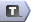# Logic in AMPL

## Relational Operators

Relational operators are used to compare two expressions. They are most commonly used in constraints, but not exclusively. The relational operators are:

Expression Meaning
< Less than
<= Less than or equal to
> Greater than
>= Greater than or equal to
>= Equal to
<> Not equal to

## Logical Operators

Logical operators are used to combine logical expressions. They are most commonly used in conditional statements, conditional structures and conditional loops. The logical operators are `not`, `and` and `or`. They are used as shown in the table below:

Expression Result
`not <expression>` True if `<expression>` is false, false if `<expression>` is true
`<e1> and <e2>` True if `<e1>` and `<e2>` are both true, otherwise false
`<e1> or <e2>` True if either `<e1>` or `<e2>` are true, otherwise false

## Logical Expressions

Logical expressions are expressions that will evaluate to either true or false. Logical expressions are usually defined in terms of the relational operators:

```Lower[r] <= sum {i in INGREDIENTS} Contributes[r, i] * Amount[i]
```

```sum {s in SURFBOARDS} Recipe[m, s] * Production[s] <= Supply[m];
```

However, there are some special logical expressions in AMPL for use with sets:

1. `<e> in <SET>` is true if `<e>` is a member of `<SET>`;
2. {\tt <e> not in <SET>} is false if `<e>` is a member of `<SET>`;
3. `exists {<e> in <SET>} <expression>` is true if some `<e>` in `<SET>` has `<expression>` being true;
4. `forall {<e> in <SET>} <expression>` is true if all `<e>` in `<SET>` have `<expression>` being true;
5. `<SUBSET> within <SET>` is true if all the elements in `<SUBSET>` are in `<SET>`;
6. `<SUBSET> not within <SET>` is true if some element in `<SUBSET>` is not in `<SET>`.

Logical expressions can be built up from other logical expressions, binary parameters and logical operators.

## Conditional Expressions

A conditional expression is very much like the `IF` function in Microsoft Excel:

```param ifvalue := if <some logical expression> then
<a value>
[else
<another value>];
```

If the logical expression is true then `ifvalue` will be set to `<a value>`, otherwise it is set to 0 (by default) or, if the else part of the expression is present, `<another value>`. Note that if the `else` keyword is present, then no `;` needs to be included after `<a value>`.

## Conditional Structures

A conditional structure is the same as the classical if_-_then_-_else statement in programming languages like MATLAB, Fortran, Visual Basic and C++:

```if <logical expression> then
<a statement>;
[else
<another statement>;]
```

Note here that even if the `else` keyword is present you need to end `<a statement>` with `;`. If you want to include more than one statement within the conditional structures you can use `{` and `}` to enclose your statements:

```if <logical expression> then
{
<some statements>
}
[else
{
<some other statements>
}]
```

## Binary Parameters

In AMPL we can create binary parameters by using the `binary` keyword in the parameter declaration:

```param stillSearching binary;
```

Binary parameters are used in a similar way to boolean variables (in Matlab, C, etc) and logical variables (Fortran). If a binary parameter has the value 0 this is equivalent to false, and 1 is equivalent to true. Binary parameters can be used with conditional expressions to hold a true/false result from a logical expression:

```param isGreater binary;

let isGreater := if 4 > 5 then 1 else 0; # isGreater = 0 (false)
let isGreater := if 6 > 5 then 1; # else 0 is the default, isGreater = 1 (true)
```
so the syntax is
```let <binary parameter> := if <expression> then 1;
```

You can also set binary parameters within conditional structures

```binary <binary parameter>;

if <expression> then
let <binary parameter> := 1;
else
let <binary parameter> := 0;
```

Binary parameters may be used in logical expressions or as the condition in a conditional statement or conditional structure. They are very useful for building complex conditional statements or structures:

```Some example from depth first searching or column generation
```
and controlling conditional loops:
```Some example from depth first searching or column generation
```

-- TWikiAdminGroup - 18 Mar 2008

Edit | Attach | Watch | Print version |  | Backlinks | Raw View | Raw edit | More topic actions...
Topic revision: r4 - 2008-03-18 - TWikiAdminUserEdit AttachCopyright © 2008-2022 by the contributing authors. All material on this collaboration platform is the property of the contributing authors.
Ideas, requests, problems regarding TWiki? Send feedback